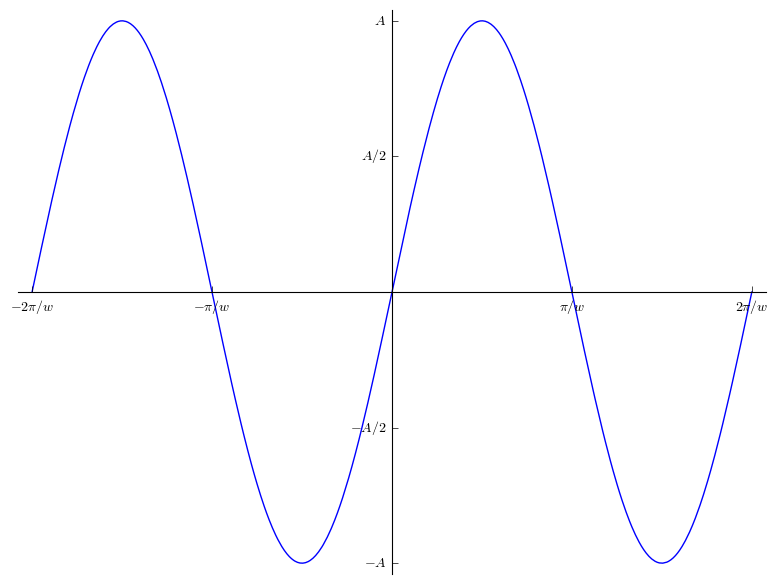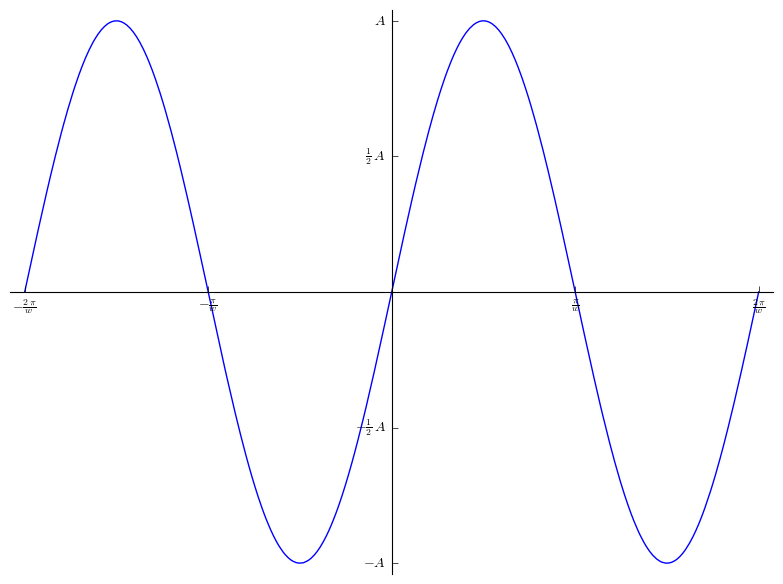# Is it possible to plot with symbols for x and y ticks?

I'd like to plot a function similar to something like: y= Asin(wx) Something along the lines of

x = var('x')

w = var('w')

A = var('A')

y = Asin(wx)

assume(w>0)

assume(A>0)

Then be able to do:

plot(y(x=x), (x,-2pi()/w,2pi()/w)

And have sage plot the function, where for the x-axis the ticks would be something like (-2pi/w, -pi/w, 0, pi/w, 2pi/w) and the y-ticks would look like (-A, -A/2,0, A/2, A).

Hopefully that makes sense? Is this possible?

edit retag close merge delete

Please give the mathematical description of the task. (To plot something, one needs to evaluate to a real number a function, so there should be no variables as obstacles for the plot around.)

So we first fix parameters $w,A$, and need a 2D-plot of the function of one variable, $x$, given by the formula $$f(x) = A\sin(wx)\ ,$$ or we fix (and give) a variable $A$, and need a $3D$-Plot of the function $$g(x,w)=A\sin(wx)\ ,$$ or something related...?!

The "ticks" are only simple marks on the coordinate axes?

Hi Dan, thanks for your reply. The first option you gave was the situation I was trying to describe: Fix parameters w, A and get a 2D-plot of the function of variable x.

And yes the tics I'm referring to are simple marks on the coordinate axes -I'm using gnuplot terminology there.

I know you could do this trivially with something like plot(y(x=x, w=2, A=5), (x,-pi(),pi())

But I was interested to see if Sage could plot it without me providing it a specific constant for A and w, but instead using the "assume" operator to define those constants as being real and positive, and plot with the axes expressed in terms of A and w rather than numerically.

Obviously this is just an example, the function I'm dealing with is a bit more complicated.

Does that clarify my question?

Sort by » oldest newest most voted

If I correctly understand your question, you want to plot some symbolic function such as $x\mapsto A \sin(wx)$, where $A$ and $w$ are symbolic variables. This is not per se possible since plotting needs floating point values. The solution consists in plotting $x\mapsto \sin(x)$ and changing the labels on the axes. The command

plot(sin(x), (x,-2*pi, 2*pi), ticks = [xt, yt], tick_formatter = [xt_lbl, yt_lbl])


plots the function $x\mapsto \sin(x)$, putting ticks on the x-axis at each location given in the list xt, and ticks on the y-axis using yt. The lists xt_lbl and yt_lbl are the labels at each tick. For instance,

sage: xt = [k * pi for k in range(-2,3)]
sage: xt_lbl = ['$-2\pi/w$', '$-\pi/w$', '$0$', '$\pi/w$', '$2\pi/w$']
sage: yt = [k / 2 for k in range(-2, 3)]
sage: yt_lbl = ['$-A$', '$-A/2$', '$0$', '$A/2$', '$A$']
sage: plot(sin(x),(x,-2*pi,2*pi), ticks=[xt, yt], tick_formatter = [xt_lbl, yt_lbl])
Launched png viewer for Graphics object consisting of 1 graphics primitive


creates the following plot:For more information on ticks and tick_formatter, please refer to the documentation on 2D-plotting (search for "ticks" in the page) and the options of show().

Addendum. You may want to automatize the process a bit more. If you accept to personalize a bit less the labels, this is possible: you can use SageMath's capabilities in the simplification of symbolic expressions, combined with the function latex which produces a LaTeX output from an expression. The following example should be self-explanatory.

sage: xt = [k * pi for k in range(-2, 3)]
sage: yt = [k/2 for k in range(-2, 3)]
sage: var('w,A')
(w, A)
sage: xt_lbl = ['$' + latex(t/w) + '$' for t in xt]
sage: yt_lbl = ['$' + latex(A*t) + '$' for t in yt]
sage: plot(sin(x),(x,-2*pi, 2*pi), ticks=[xt, yt], tick_formatter=[xt_lbl, yt_lbl])
Launched png viewer for Graphics object consisting of 1 graphics primitivemore

Thanks Bruno, this is exactly what I want to do. However the function I wish to plot is a bit more complicated than just sin(x) so I was hoping that there would be a way to get sage to figure out where to put the symbolic ticks.

But as you say, if plot requires floating point numbers, then sage is probably not capable of automagically doing the symbolic plotting I'm after.

I think it would be a neat capability to add though!